NetworkX

degrees

#### Next topic

projected_graph

biadjacency_matrix(G, row_order, column_order=None, weight='weight', dtype=None)[source]

Return the biadjacency matrix of the bipartite graph G.

Let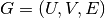be a bipartite graph with node sets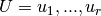and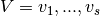. The biadjacency matrix  is the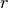x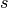matrix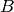in which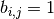if, and only if,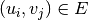. If the parameter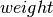is not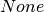and matches the name of an edge attribute, its value is used instead of 1.

Parameters : G : graph A NetworkX graph row_order : list of nodes The rows of the matrix are ordered according to the list of nodes. column_order : list, optional The columns of the matrix are ordered according to the list of nodes. If column_order is None, then the ordering of columns is arbitrary. weight : string or None, optional (default=’weight’) The edge data key used to provide each value in the matrix. If None, then each edge has weight 1. dtype : NumPy data type, optional A valid single NumPy data type used to initialize the array. This must be a simple type such as int or numpy.float64 and not a compound data type (see to_numpy_recarray) If None, then the NumPy default is used. B : numpy matrix Biadjacency matrix representation of the bipartite graph G.